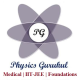# MCQ Questions for Class 10 Science Light Reflection and Refraction with Answers

Home » CBSE Class 10 Science » MCQ Questions for Class 10 Science » MCQ Questions for Class 10 Science Light Reflection and Refraction with Answers

Question 1. If an incident ray passes through the focus, the reflected ray will
(a) pass through the pole
(b) be parallel to the principal axis
(c) retrace its path
(d) pass through the centre of curvature

Question 2. When light falls on a smooth polished surface, most of it
(a) is reflected in the same direction
(b) is reflected in different directions
(c) is scattered
(d) is refracted into the second medium

Question 3. Image formed by reflection from a plane mirror is
(a) real and inverted
(b) virtual and erect
(c) real and erect
(d) virtual and inverted

Question 4. Magnifying power of a concave lens is
(a) always > 1
(b) always < 1
(c) always = 1
(d) can have any value

Question 5. The image formed by a convex lens can be
(a) virtual and magnified
(b) virtual and diminished
(c) virtual and of same size
(d) virtual image is not formed

Question 6. Focal length of a concave mirror is
(a) negative
(b) positive
(c) depends on the position of object
(d) depends on the position of image

Question 7. If the power of a lens is – 2 D, what is its focal length?
(a) +50 cm
(b) -100 cm
(c) -50 cm
(d) +100 cm

Question 8. A spherical mirror and a spherical lens each have a focal length of -10 cm. The mirror and the lens are likely to be
(a) both concave
(b) both convex
(c) the mirror is concave and the lens is convex
(d) the mirror is convex and the lens is concave

Question 9. If the magnification produced by a lens has a negative value, the image will be
(a) virtual and inverted
(b) virtual and erect
(c) real and erect
(d) real and inverted

Question 10. A point object is placed at a distance of 20 cm from a convex mirror of focal length 20 cm. The image will form at:
(a) at infinity
(b) at focus
(c) at the pole
(d) behind the mirror

Question 11. When the object is placed between f and 2f of a convex lens, the image formed is
(a) at f
(b) at 2f
(c) beyond 2f
(d) between O and f

Question 12. Which mirroji can produce a virtual, erect and magnified ifhage of an object?
(a) Concave mirror
(b) Convex mirror
(c) Plane mirror
(d) Both concave and convex mirrors

Question 13. If the image is formed in front of the mirror, then the image distance will be
(a) positive or negative depending on the size of the object
(b) neither positive nor negative
(c) positive
(d) negative

Question 14. A ray of light is travelling from a rarer medium to a denser medium. While entering the denser medium at the point of incidence, it
(a) goes straight into the second medium
(b) bends towards the normal
(c) bends away from the normal
(d) does not enter at all

Question 15. A student does the experiment on tracing the path of a ray of light passing through a rectangular glass slab for different angles of incidence. He can get a correct measure of the angle of incidence and the angle of emergence by following the labelling indicated in figure:

(a) I
(b) II
(c) III
(d) IV

Fill in the Blanks:

1. Light shows the phenomena of reflection, refraction and ………. .
2. The speed of light in vacuum is ………. .
3. Power of’ a lens is the ………. of its focal length.
4. The SI unit of power is ………. .
5. A ………. lens will always give a virtual, erect and diminished image, irrespective of the position of the object.
6. A positive sign in the value of magnification indicates that the image is ………. .
7. A ………. mirror is used as a head mirror by the doctors to concentrate light on the body parts to be examined.
8. No matter how far you stand from a spherical mirror, your image appears erect. The mirror may be ………. .

1. dispersion
2. 3 x 108 m/s
3. reciprocal
4. dioptre
5. concave
6. virtual
7. concave
8. plane or convex mirror.

One App For All Your Needs.

Watch this lecture for better understanding of Respiration.

Learn about Respiration, Breathing and Respiration, Difference between Breathing and Respiration, How energy released during respiration is stored, Types of Respiration, Aerobic Respiration, Anaerobic Respiration

Respiration- The process of releasing energy from food is called respiration. The process of respiration involves taking in oxygen (of air) into the cells, using it for releasing energy by burning food, and then eliminating the waste products (carbon dioxide and water) from the body……….(Read more)

## 6 thoughts on “MCQ Questions for Class 10 Science Light Reflection and Refraction with Answers”

1.Where can we see the answers ?

1.2.